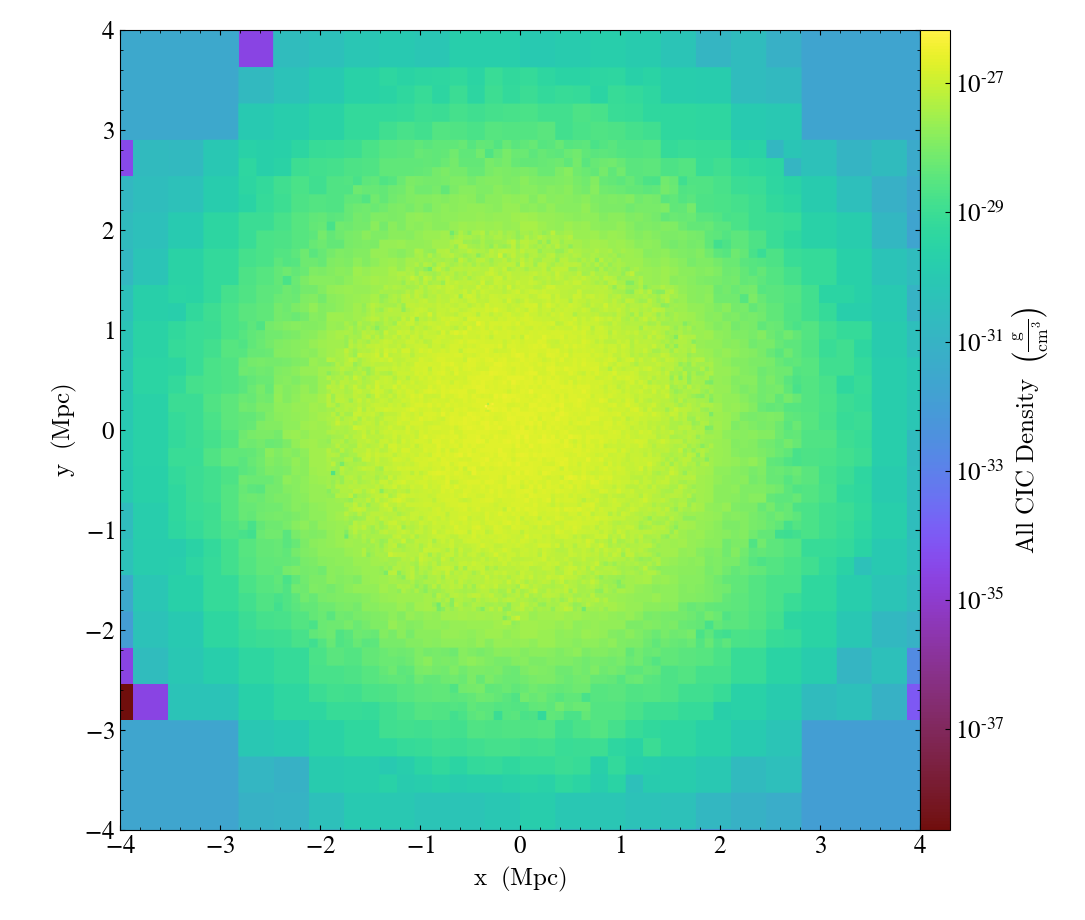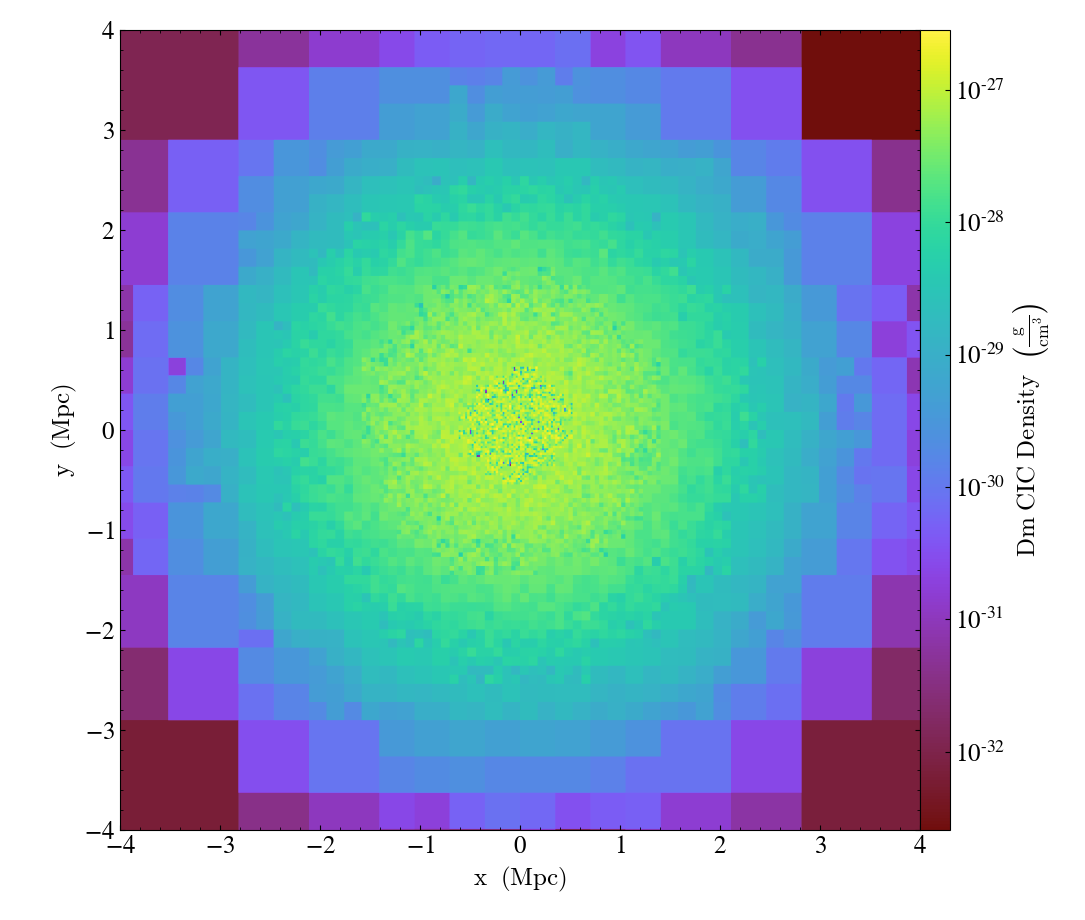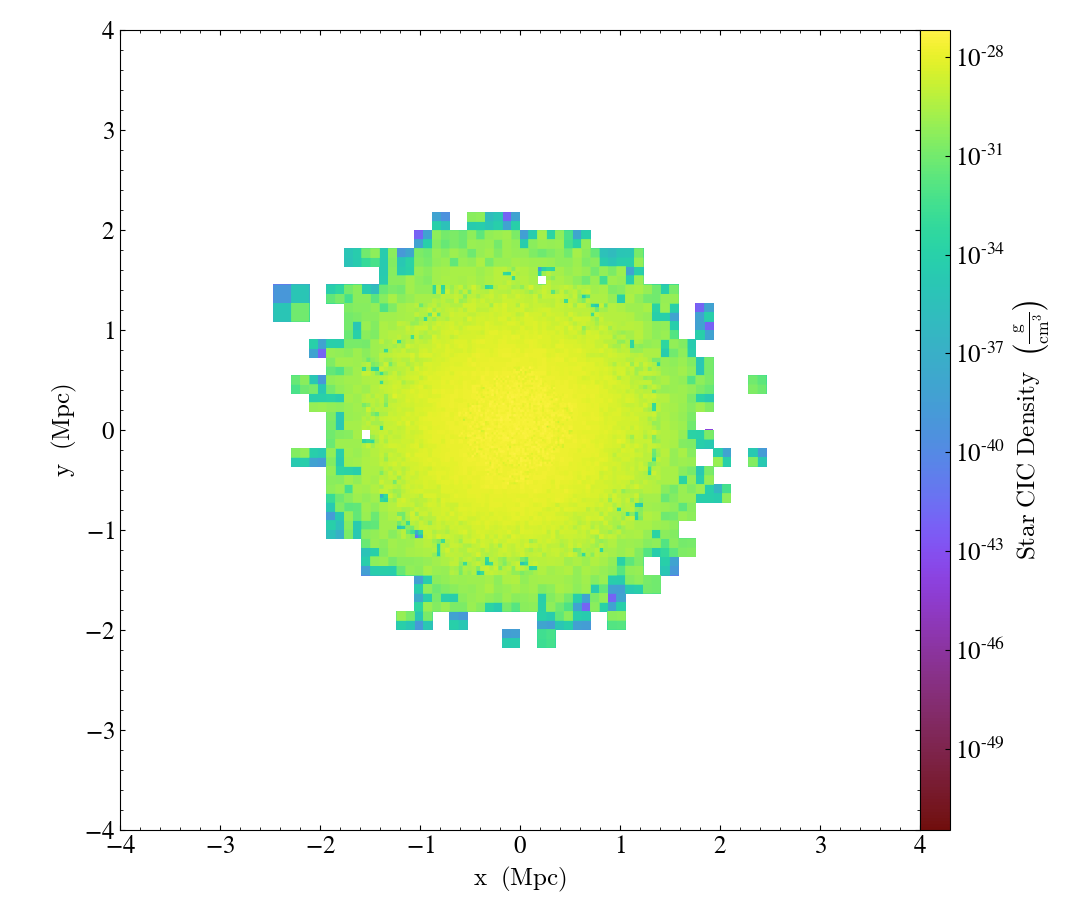# Loading Generic Particle Data¶

Notebook

This example creates a fake in-memory particle dataset and then loads it as a yt dataset using the load_particles function.

Our "fake" dataset will be numpy arrays filled with normally distributed randoml particle positions and uniform particle masses. Since real data is often scaled, I arbitrarily multiply by 1e6 to show how to deal with scaled data.

In :
import numpy as np

n_particles = 5000000

ppx, ppy, ppz = 1e6*np.random.normal(size=[3, n_particles])

ppm = np.ones(n_particles)


The load_particles function accepts a dictionary populated with particle data fields loaded in memory as numpy arrays or python lists:

In :
data = {'particle_position_x': ppx,
'particle_position_y': ppy,
'particle_position_z': ppz,
'particle_mass': ppm}


To hook up with yt's internal field system, the dictionary keys must be 'particle_position_x', 'particle_position_y', 'particle_position_z', and 'particle_mass', as well as any other particle field provided by one of the particle frontends.

The load_particles function transforms the data dictionary into an in-memory yt Dataset object, providing an interface for further analysis with yt. The example below illustrates how to load the data dictionary we created above.

In :
import yt
from yt.units import parsec, Msun

bbox = 1.1*np.array([[min(ppx), max(ppx)], [min(ppy), max(ppy)], [min(ppz), max(ppz)]])

ds = yt.load_particles(data, length_unit=parsec, mass_unit=1e8*Msun, n_ref=256, bbox=bbox)


The length_unit and mass_unit are the conversion from the units used in the data dictionary to CGS. I've arbitrarily chosen one parsec and 10^8 Msun for this example.

The n_ref parameter controls how many particle it takes to accumulate in an oct-tree cell to trigger refinement. Larger n_ref will decrease poisson noise at the cost of resolution in the octree.

Finally, the bbox parameter is a bounding box in the units of the dataset that contains all of the particles. This is used to set the size of the base octree block.

This new dataset acts like any other yt Dataset object, and can be used to create data objects and query for yt fields. This example shows how to access "deposit" fields:

In :
ad = ds.all_data()

# This is generated with "cloud-in-cell" interpolation.
cic_density = ad["deposit", "all_cic"]

# These three are based on nearest-neighbor cell deposition
nn_density = ad["deposit", "all_density"]
nn_deposited_mass = ad["deposit", "all_mass"]
particle_count_per_cell = ad["deposit", "all_count"]

In :
ds.field_list

Out:
[('all', 'particle_mass'),
('all', 'particle_position_x'),
('all', 'particle_position_y'),
('all', 'particle_position_z'),
('io', 'particle_mass'),
('io', 'particle_position_x'),
('io', 'particle_position_y'),
('io', 'particle_position_z')]
In :
ds.derived_field_list

Out:
[('all', 'mesh_id'),
('all', 'particle_mass'),
('all', 'particle_ones'),
('all', 'particle_position'),
('all', 'particle_position_cylindrical_radius'),
('all', 'particle_position_cylindrical_theta'),
('all', 'particle_position_cylindrical_z'),
('all', 'particle_position_relative'),
('all', 'particle_position_relative_x'),
('all', 'particle_position_relative_y'),
('all', 'particle_position_relative_z'),
('all', 'particle_position_spherical_phi'),
('all', 'particle_position_spherical_radius'),
('all', 'particle_position_spherical_theta'),
('all', 'particle_position_x'),
('all', 'particle_position_y'),
('all', 'particle_position_z'),
('all', 'particle_radius'),
('all', 'particle_spherical_position_phi'),
('all', 'particle_spherical_position_radius'),
('all', 'particle_spherical_position_theta'),
('all', 'relative_particle_position'),
('all', 'relative_particle_position_x'),
('all', 'relative_particle_position_y'),
('all', 'relative_particle_position_z'),
('deposit', 'all_cic'),
('deposit', 'all_count'),
('deposit', 'all_density'),
('deposit', 'all_mass'),
('deposit', 'io_cic'),
('deposit', 'io_count'),
('deposit', 'io_density'),
('deposit', 'io_mass'),
('gas', 'cell_volume'),
('gas', 'dx'),
('gas', 'dy'),
('gas', 'dz'),
('gas', 'path_element_x'),
('gas', 'path_element_y'),
('gas', 'path_element_z'),
('gas', 'vertex_x'),
('gas', 'vertex_y'),
('gas', 'vertex_z'),
('gas', 'x'),
('gas', 'y'),
('gas', 'z'),
('index', 'cell_volume'),
('index', 'cylindrical_r'),
('index', 'cylindrical_radius'),
('index', 'cylindrical_theta'),
('index', 'cylindrical_z'),
('index', 'disk_angle'),
('index', 'dx'),
('index', 'dy'),
('index', 'dz'),
('index', 'grid_indices'),
('index', 'grid_level'),
('index', 'height'),
('index', 'morton_index'),
('index', 'ones'),
('index', 'ones_over_dx'),
('index', 'path_element_x'),
('index', 'path_element_y'),
('index', 'path_element_z'),
('index', 'radius'),
('index', 'spherical_phi'),
('index', 'spherical_r'),
('index', 'spherical_radius'),
('index', 'spherical_theta'),
('index', 'vertex_x'),
('index', 'vertex_y'),
('index', 'vertex_z'),
('index', 'virial_radius_fraction'),
('index', 'x'),
('index', 'y'),
('index', 'z'),
('index', 'zeros'),
('io', 'mesh_id'),
('io', 'particle_mass'),
('io', 'particle_ones'),
('io', 'particle_position'),
('io', 'particle_position_cylindrical_radius'),
('io', 'particle_position_cylindrical_theta'),
('io', 'particle_position_cylindrical_z'),
('io', 'particle_position_relative'),
('io', 'particle_position_relative_x'),
('io', 'particle_position_relative_y'),
('io', 'particle_position_relative_z'),
('io', 'particle_position_spherical_phi'),
('io', 'particle_position_spherical_radius'),
('io', 'particle_position_spherical_theta'),
('io', 'particle_position_x'),
('io', 'particle_position_y'),
('io', 'particle_position_z'),
('io', 'particle_radius'),
('io', 'particle_spherical_position_phi'),
('io', 'particle_spherical_position_radius'),
('io', 'particle_spherical_position_theta'),
('io', 'relative_particle_position'),
('io', 'relative_particle_position_x'),
('io', 'relative_particle_position_y'),
('io', 'relative_particle_position_z'),
('stream', 'cell_volume'),
('stream', 'dx'),
('stream', 'dy'),
('stream', 'dz'),
('stream', 'path_element_x'),
('stream', 'path_element_y'),
('stream', 'path_element_z'),
('stream', 'vertex_x'),
('stream', 'vertex_y'),
('stream', 'vertex_z'),
('stream', 'x'),
('stream', 'y'),
('stream', 'z')]
In :
slc = yt.SlicePlot(ds, 2, ('deposit', 'all_cic'))
slc.set_width((8, 'Mpc'))

Out:Finally, one can specify multiple particle types in the data directory by setting the field names to be field tuples (the default field type for particles is "io") if one is not specified:

In :
n_star_particles = 1000000
n_dm_particles = 2000000

ppxd, ppyd, ppzd = 1e6*np.random.normal(size=[3, n_dm_particles])
ppmd = np.ones(n_dm_particles)

ppxs, ppys, ppzs = 5e5*np.random.normal(size=[3, n_star_particles])
ppms = 0.1*np.ones(n_star_particles)

data2 = {('dm', 'particle_position_x'): ppxd,
('dm', 'particle_position_y'): ppyd,
('dm', 'particle_position_z'): ppzd,
('dm', 'particle_mass'): ppmd,
('star', 'particle_position_x'): ppxs,
('star', 'particle_position_y'): ppys,
('star', 'particle_position_z'): ppzs,
('star', 'particle_mass'): ppms}

ds2 = yt.load_particles(data2, length_unit=parsec, mass_unit=1e8*Msun, n_ref=256, bbox=bbox)


We now have separate "dm" and "star" particles, as well as their deposited fields:

In :
slc = yt.SlicePlot(ds2, 2, [('deposit', 'dm_cic'), ('deposit', 'star_cic')])
slc.set_width((8, 'Mpc'))

Out: